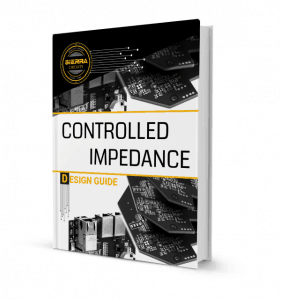## How to Analyze a PCB Transmission Line?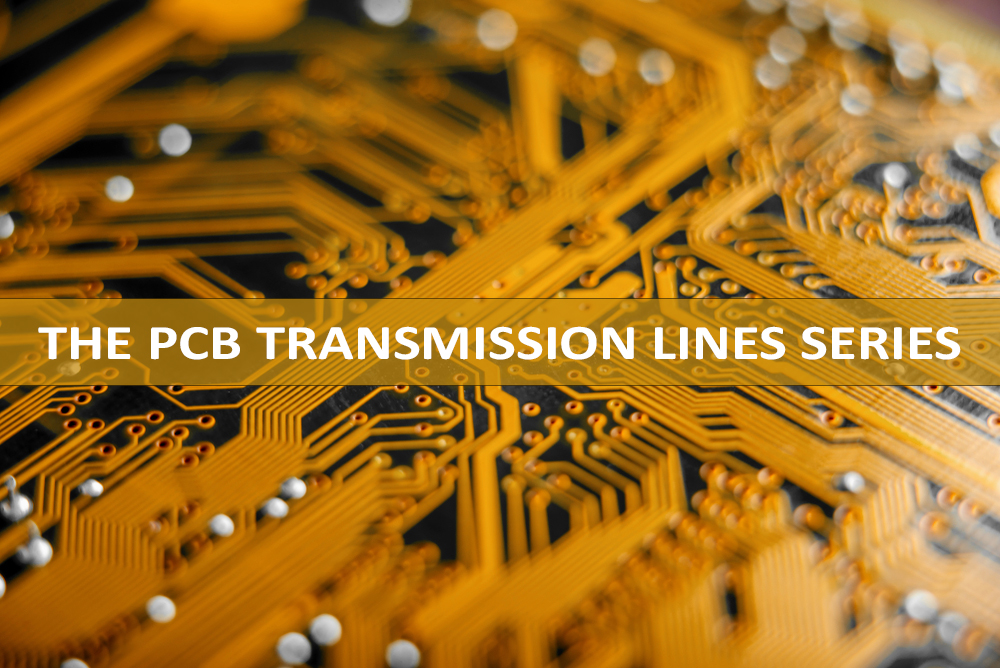After establishing what a PCB transmission line is and talking about signal speed and propagation delay, and the critical length, the controlled impedance, and the rise/fall time of a PCB transmission line, we discussed impedance discontinuity and signal reflection. Today, we are going to explain how to analyze a PCB transmission line using quantities, such as voltages and currents, and line parameters, such as resistance, inductance, capacitance, and conductance.

Basically, high-speed or high-frequency signals generate electromagnetic fields around them during their travel along a transmission line, and their behavior is best analyzed using Maxwell’s electromagnetic equations and the theory of electromagnetic wave propagation. In this method, we have to deal with electric and magnetic fields instead of usual voltages and currents. The voltage between the signal line and its return path will generate an electric field and a current in the conductors, which in turn will create a magnetic field around them. Thus, voltage, current, and electric and magnetic fields all travel as waves along the transmission line.

There exists a close analogy between the electromagnetic waves propagation and the propagation of voltages and currents along a transmission line. As it is easier to think in terms of familiar voltages and currents rather than electric and magnetic fields, we should use the voltage/current analysis of the transmission line in the following treatment.

A transmission line is a large number of infinitesimal length segments, and each segment can be analyzed by network theory concepts at a particular point in space and time, ignoring the travel time in the infinitesimal segment as its length is extremely small.

In this analysis technique, we will be dealing with quantities like voltages and currents, and line parameters like resistance, inductance, capacitance, and conductance. We will model an infinitesimal segment of a PCB transmission line in terms of the following parameters of the transmission line:

R = the resistance of the transmission line per unit length (or p.u.l.) (R in Ohms p.u.l.)
L = the inductance of the transmission line p.u.l. (L in Henrys p.u.l.)
C = the capacitance of the transmission line p.u.l. (C in Farads p.u.l.)
G = the conductance of the transmission line p.u.l. (G in Mhos p.u.l.)

We can denote the length of the infinitesimal transmission line by Δx.

Then we can picture this transmission line segment as follows:Where:
V(x,t) = signal voltage at location x at time t
I(x,t) = signal current at location x at time t

Let’s do the analysis of this circuit in the frequency domain. Here, we assume that the signals vary with time sinusoidally with an angular frequency ω, so that the time-varying part of V(x,t) and I(x,t) can be shown by the factor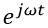and we now have: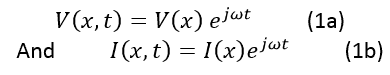Using Kirchhoff’s laws on the above segment, we obtain the following relations: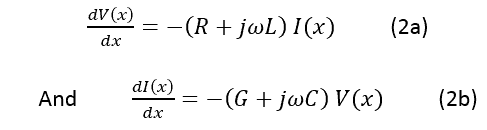And from these, by differentiation, we get: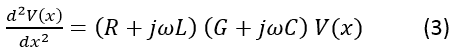A solution of this equation is: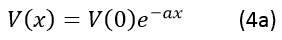And from Equation 2,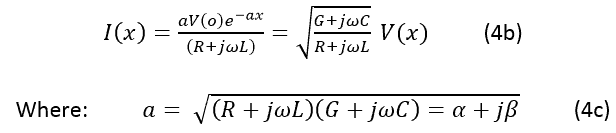α has units of p.u.l.

If we multiply Equation 4a byto reincorporate the time variations of the sinusoidal voltages and currents, we will see that now the equations represent voltage and current signal waves traveling in the positive x direction over the transmission line: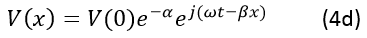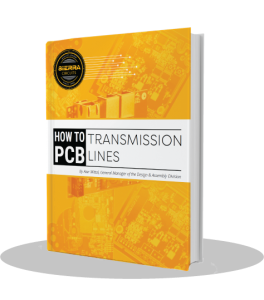## The characteristic impedance of a uniform transmission line

From the above Equations 4, we will obtain a relationship between V(x) and I(x) as follows: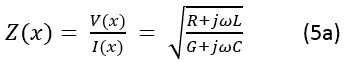The parameters R, L, G and C depend on the geometry (shape, width, etc.) of the relevant PCB conductors forming the transmission line and the properties of the conductors and dielectric materials used in the PCB.

If the material and the geometrical properties are assumed to be uniform along the length of the transmission line, and the PCB materials are considered homogeneous, then R, L, G and C have the same value at every location along the length of the transmission line. This means that the above impedance has the same value for all values of x along the transmission line. This kind of transmission line is called a uniform transmission line and its impedance is: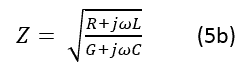This is the characteristic impedance of the uniform transmission line and it is its most important property from the signal integrity perspective. In the PCB industry, we generally refer to characteristic impedance as just “the impedance” of the transmission line.

If the PCB manufacturing process is such that we are able to control the geometry of the PCB transmission lines within a specified tolerance range, then we could obtain the impedance value of the PCB transmission line at every location along its length within a specified tolerance of a desired value. This way, the PCB transmission line has a controlled impedance and is called a controlled impedance PCB.

If we look at an infinite transmission line of characteristic impedance Z0 from the left side at any point, we can see an impedance of Z0. Therefore, if we take a finite length transmission line of impedance Z0 and terminate it on the right by an impedance of value Z0, and if we look at the finite transmission line from the left, it will appear as an infinite transmission of impedance Z0 from the impedance perspective: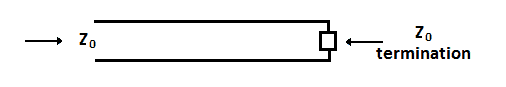## Lossless uniform transmission line

As the equation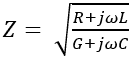shows, the impedance Z is a function of the signal frequency ω.

Practically speaking, R << ωL and G << ωC at signal frequencies of our interest. If we ignore R and G in this formulation, we get a lossless transmission line.

The impedance of a lossless uniform transmission line is given by: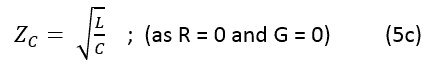The impedance of a lossless transmission line is no longer a direct function of frequency, which means it has the same value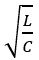at all signal frequencies. Note thathas units of Ohms.

In a lossless transmission line (R = 0, G = 0),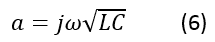The voltages and currents, taking into account of Equations 4, are given by: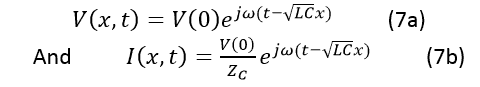These relations represent voltage and current waves traveling in the positive x direction with a speed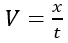and the propagation delay tpd per unit length given by: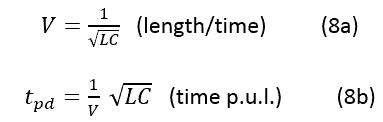## Estimation of L and C if Zc and tpd are known

The parameters V and tpd depend on the dielectric constant of the PCB material and these parameters can be calculated separately as we have described in our article about signal speed and propagation delay in a transmission line. Therefore, if we know tpd and Zc, we can calculate L and C per unit length values from the relations Equation 5 and Equation 8 as follows:Example:

For a microstrip transmission line in a PCB material of FR4 having Er = 4, if Zc = 50 ohms and tpd = 145 psec/inch, we get:### Summary

A transmission line comprises of at least two conductors – one for the signal and another for the signals’ return path.

At high-speed or high-frequency signals, we can’t ignore the transmission line effects. On a transmission line, time-varying electrical signals – voltages and currents – propagate as waves – accompanied by analogous wave propagation of their electrical and magnetic fields.

For a uniform transmission line, we investigated its two most important parameters – its characteristic impedance and signal velocity/propagation delay.

Given a digital signals’ rise/fall time or clock frequency or data transfer rate or the maximum frequency of an analog signal, and the dielectric constant of the material (or the medium) in which the line exists, we defined a concept called critical length lc which clearly helps us in a real-life situation as to whether we should design the PCB interconnects as controlled impedance transmission lines or not.

#### Read the previous articles of our PCB Transmission Line series: